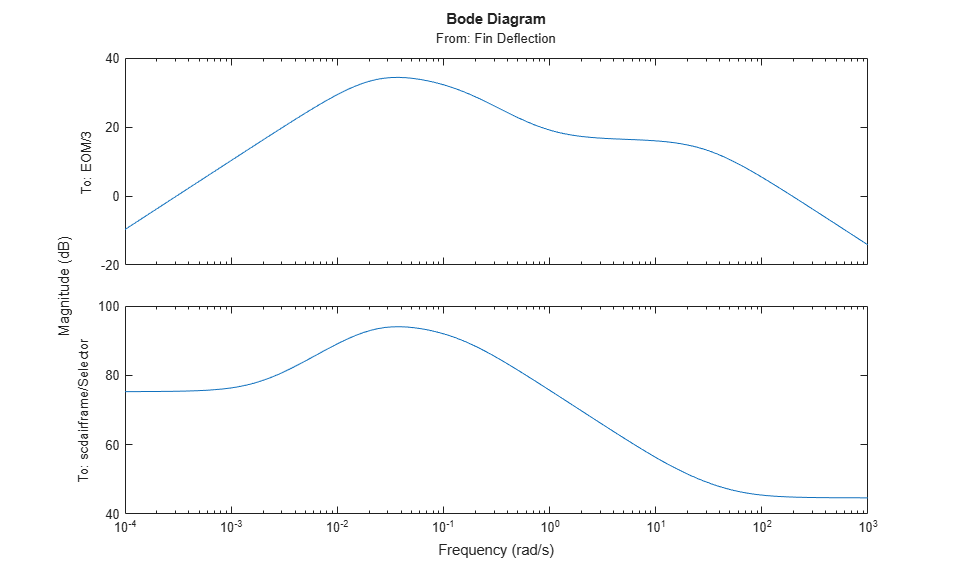Trim and Linearize an Airframe

This example shows how to trim and linearize an airframe using Simulink® Control Design™ software.

The goal is to find the elevator deflection and the resulting trimmed body rate that generate a given angle of incidence when the airframe is traveling at a set speed.

Once you find the trim condition, you can compute a linear model for the dynamics of the states around the trim condition.

Fixed parameters:

• Angle of incidence (Theta)

• Body attitude (U)

• Position

• Elevator deflection (w)

• Body rate (q)

Compute Operating Points

Open the model.

mdl = 'scdairframe';
open_system(mdl)Create an operating point specification object for the model using the model initial conditions.

opspec = operspec(mdl)
opspec =

Operating point specification for the Model scdairframe.
(Time-Varying Components Evaluated at time t=0)

States:
----------
<strong>x</strong>      <strong>Known</strong>    <strong>SteadyState</strong>    <strong>Min</strong>     <strong>Max</strong>    <strong>dxMin</strong>    <strong>dxMax</strong>
<strong>_____</strong>    <strong>_____</strong>    <strong>___________</strong>    <strong>____</strong>    <strong>___</strong>    <strong>_____</strong>    <strong>_____</strong>

(1.) scdairframe/EOM/ Equations of Motion (Body Axes)/Position
0    false       true        -Inf    Inf    -Inf      Inf
-3048    false       true        -Inf    Inf    -Inf      Inf
(2.) scdairframe/EOM/ Equations of Motion (Body Axes)/Theta
0    false       true        -Inf    Inf    -Inf      Inf
(3.) scdairframe/EOM/ Equations of Motion (Body Axes)/U,w
984    false       true        -Inf    Inf    -Inf      Inf
0    false       true        -Inf    Inf    -Inf      Inf
(4.) scdairframe/EOM/ Equations of Motion (Body Axes)/q
0    false       true        -Inf    Inf    -Inf      Inf

Inputs:
----------
<strong>u</strong>    <strong>Known</strong>    <strong>Min</strong>     <strong>Max</strong>
<strong>_</strong>    <strong>_____</strong>    <strong>____</strong>    <strong>___</strong>

(1.) scdairframe/Fin Deflection
0    false    -Inf    Inf

Outputs:
----------
<strong>y</strong>    <strong>Known</strong>    <strong>Min</strong>     <strong>Max</strong>
<strong>_</strong>    <strong>_____</strong>    <strong>____</strong>    <strong>___</strong>

(1.) scdairframe/q
0    false    -Inf    Inf
(2.) scdairframe/az
0    false    -Inf    Inf

Specify which states in the model are:

• Known at the operating point

• At steady state at the operating point

Specify that the Position states are known and not at steady state. For the state values, specified in opspec.States(1).x, use the default values from the model initial condition.

opspec.States(1).Known = [1;1];

Specify that the second state, which corresponds to the angle of incidence Theta, is known but not at steady state. As with the position states, use the default state value from the model initial condition.

opspec.States(2).Known = 1;

The third state specification includes the body axis angular rates U and w. Specify that both states are known at the operating point and that w is at steady state.

opspec.States(3).Known = [1 1];

Search for the operating point that meets these specifications.

op = findop(mdl,opspec);
Operating point search report:
---------------------------------

opreport =

Operating point search report for the Model scdairframe.
(Time-Varying Components Evaluated at time t=0)

Operating point specifications were successfully met.
States:
----------
<strong>Min</strong>         <strong>x</strong>          <strong>Max</strong>     <strong>dxMin</strong>        <strong>dx</strong>         <strong>dxMax</strong>
<strong>_____</strong>    <strong>__________</strong>    <strong>_____</strong>    <strong>_____</strong>    <strong>___________</strong>    <strong>_____</strong>

(1.) scdairframe/EOM/ Equations of Motion (Body Axes)/Position
0             0        0    -Inf             984     Inf
-3048         -3048    -3048    -Inf               0     Inf
(2.) scdairframe/EOM/ Equations of Motion (Body Axes)/Theta
0             0        0    -Inf      -0.0097235     Inf
(3.) scdairframe/EOM/ Equations of Motion (Body Axes)/U,w
984           984      984    -Inf           22.69     Inf
0             0        0       0     -1.4367e-11       0
(4.) scdairframe/EOM/ Equations of Motion (Body Axes)/q
-Inf    -0.0097235      Inf       0      1.1477e-16       0

Inputs:
----------
<strong>Min</strong>         <strong>u</strong>        <strong>Max</strong>
<strong>____</strong>    <strong>_________</strong>    <strong>___</strong>

(1.) scdairframe/Fin Deflection
-Inf    0.0014161    Inf

Outputs:
----------
<strong>Min</strong>         <strong>y</strong>         <strong>Max</strong>
<strong>____</strong>    <strong>__________</strong>    <strong>___</strong>

(1.) scdairframe/q
-Inf    -0.0097235    Inf
(2.) scdairframe/az
-Inf      -0.24207    Inf

Linearize Model

To linearize the model at the computed operating point, first specify the linearization input and output points.

io(1) = linio('scdairframe/Fin Deflection',1,'input');
io(2) = linio('scdairframe/EOM',3,'output');
io(3) = linio('scdairframe/Selector',1,'output');

Linearize the model at the operating point.

sys = linearize(mdl,op,io);

Plot the Bode magnitude response for the linear model.

bodemag(sys)

bdclose('scdairframe')